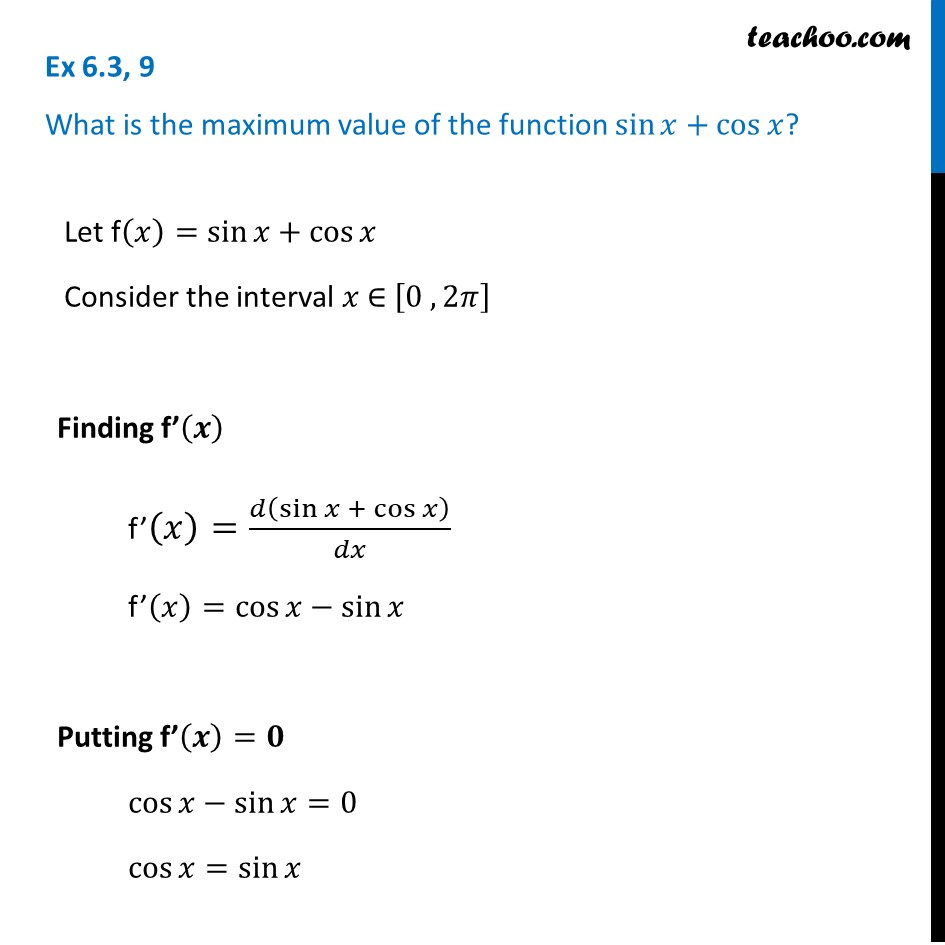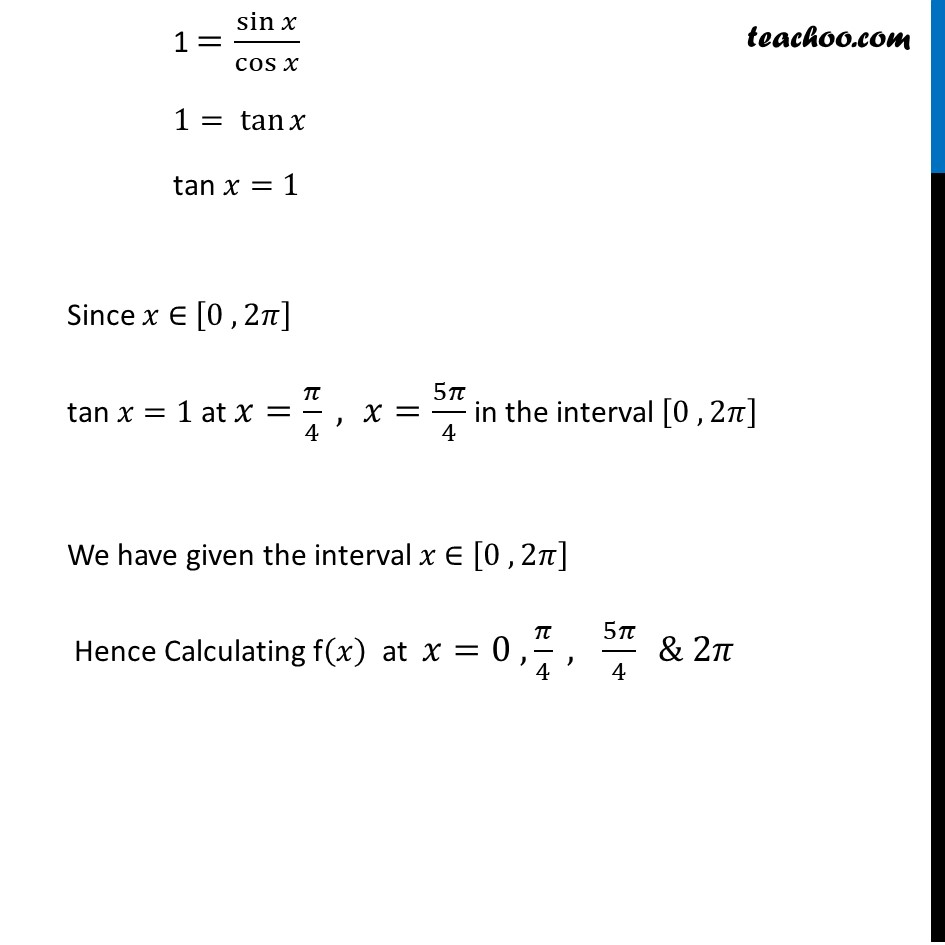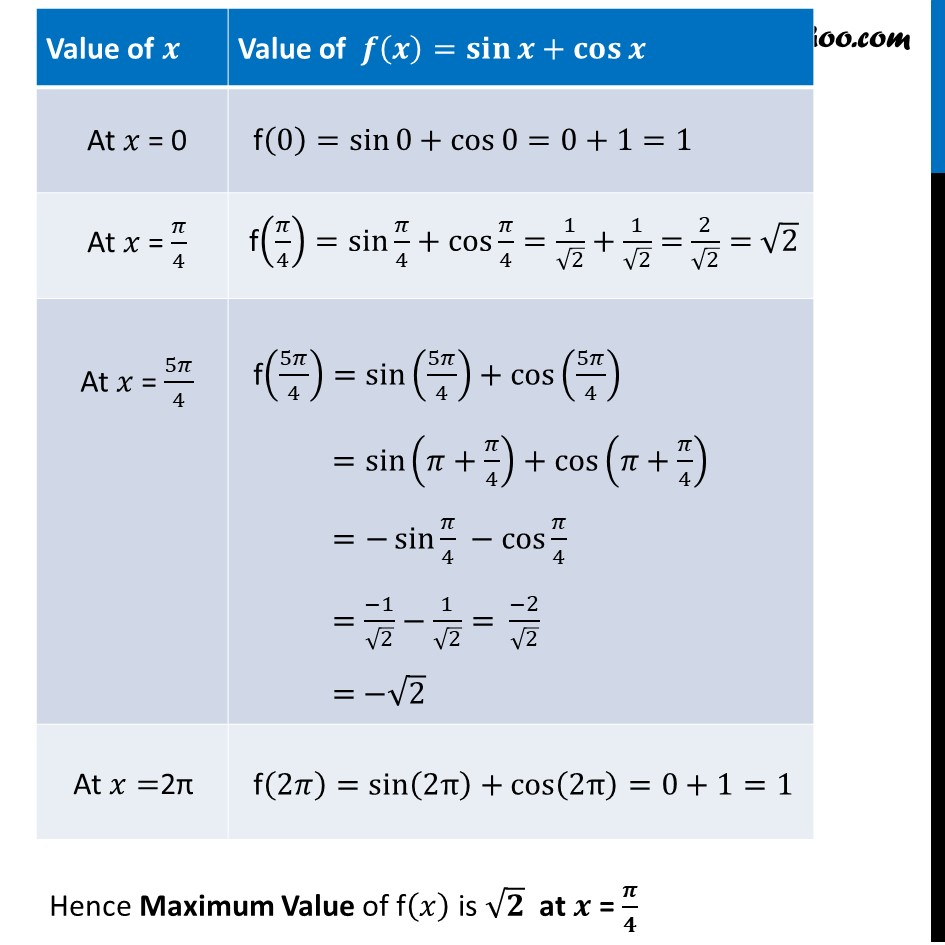Ex 6.3

Chapter 6 Class 12 Application of Derivatives
Serial order wiseLearn in your speed, with individual attention - Teachoo Maths 1-on-1 Class

### Transcript

Ex 6.3, 9 What is the maximum value of the function sin⁡𝑥+cos⁡𝑥? Let f(𝑥)=sin⁡𝑥+cos⁡𝑥 Consider the interval 𝑥 ∈ [0 , 2𝜋] Finding f’(𝒙) f’(𝑥)=𝑑(sin⁡𝑥 + cos⁡𝑥 )/𝑑𝑥 f’(𝑥)=cos⁡𝑥−sin⁡𝑥 Putting f’(𝒙)=𝟎 cos⁡𝑥−sin⁡𝑥=0 cos⁡𝑥=sin⁡𝑥 1 =sin⁡𝑥/cos⁡𝑥 1= tan⁡𝑥 tan 𝑥=1 Since 𝑥 ∈ [0 , 2𝜋] tan 𝑥=1 at 𝑥=𝜋/4 , 𝑥=5𝜋/4 in the interval [0 , 2𝜋] We have given the interval 𝑥 ∈ [0 , 2𝜋] Hence Calculating f(𝑥) at 𝑥=0 ,𝜋/4 , 5𝜋/4 & 2𝜋 Hence Maximum Value of f(𝑥) is √𝟐 at 𝒙 = 𝝅/𝟒